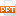# Chapter 3: Series - Module 1: Series

2.Some test: The Test for Divergent The Comparison test. The Limit Comparison test. The Alternating Series test. The Ration test. The Root test.13 trang | Chia sẻ: vutrong32 | Ngày: 20/10/2018 | Lượt xem: 120 | Lượt tải: 0
Bạn đang xem nội dung tài liệu Chapter 3: Series - Module 1: Series, để tải tài liệu về máy bạn click vào nút DOWNLOAD ở trên
Module 1:SeriesDuy Tân UniversityLecturer: Thân Thị Quỳnh DaoNatural Sciences DepartmentChapter 3: Series Module 1: Series 1. Definition Let be an infinite sequence.Then, 1. Definition Let be an infinite sequence.Then, Let:1. Definition If the sequence is convergent and exists as a real number, then the series is called convergent and we writeThe number s is called the sum of the series. Otherwise, the series is called divergent.Example:Are the following series convergent or divergent?Steps to determine the convergence or divergence of series:- Caculate sn- Find lim sn+ lim sn = s + lim sn = + Don’t exist the limit of sn- Determine anSpecial series:: geometric series.: p- series.2.Some test:The Test for DivergentThe Comparison test.The Limit Comparison test. The Alternating Series test. The Ration test. The Root test.2.Some test:a. The Test for Divergence. Let . If or does not exist then the series is divergent2.Some tests:b. The Comparison test. Positive serie: is called positive serie if 2.Some tests: The Comparison test: Let are positive series. If then, either both convergent or both divergent. b. The Comparison test.2.Some tests:b. The Comparison test. Some special series:: geometric series: p- seriesConvergent if Divergent if Convergent if Divergent if 2.Some test:c. The Ratio test. Let : and If then divergent. If then convergent.

Các file đính kèm theo tài liệu này:

•series_6187.ppt
Tài liệu liên quan